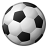QlikView App Dev

Discussion Board for collaboration related to QlikView App Development.

Announcements
QlikWorld 2022, LIVE in Denver CO., May 16-19, 2022. REGISTER NOW TO RECEIVE EARLY BIRD PRICING
cancel
Showing results for
Did you mean:Contributor II

Calculate due date based on payment terms

Hi,

I'm trying to calculate due date for invoices based on payment terms. We have 68 different payment terms with different formulas for each. As suggested in an older thread

https://community.qlik.com/message/873768#873768

I have managed to store each formula as a variable with the same name as the payment term in my data file. However I can't match the variable with my data field. Could someone please help with the syntax or other suggestion?

Invoices:

%InvID,
[Document Date]
PTCode,
Date([Document Date]+1) as duda, //Example formula

\$(PT01) as [Due Date 1], //Fixed variable works
Evaluate('\$(' & PTCode & ')') as [Due Date 2] //Getting correct variable from PTCode field does not work
FROM
Data source

Thanks

1 Solution

Accepted SolutionsContributor II
Author

Hi

If anyone has the same problem as I did I finally found a solution through this old thread:

Inside field calculation?

Variables:
PTCode, Formula
PT01, Date('DocDate'+1)
PT02, Date('DocDate'+2)
PT03, Date('DocDate'+3)
PT04, Date('DocDate'+4)
PT05, Date('DocDate'+5)
]
;

For vRowNo = 0 to NoOfRows('Variables')-1
Let vVariableName = Peek('PTCode',vRowNo,'Variables');
Let \$(vVariableName) = Peek('Formula',vRowNo,'Variables');
Next vRowNo

Drop table Variables;

Data:
[Document Date] as DocDate,

Evaluate(PTCode) as Calc,
PTCode
FROM
Invoice data.xlsx
(
ooxml, embedded labels, table is Sheet1);

LET vCalcs = repeat('replace(',nooffields('Data')) & 'Calc';

FOR I = 1 TO nooffields('Data')
LET vCalcs = vCalcs & ',' & chr(39) & fieldname(I,'Data') & chr(39) & ',' & fieldname(I,'Data') & ')';
NEXT

LEFT JOIN (Data)
ID
,
\$(vCalcs) as VarForm
,
evaluate(\$(vCalcs)) as Result
RESIDENT Data
;

11 RepliesChampion II

you need to evaluate the expressions in the front end in a chart not in the scriptContributor III

I did not think you need the single quote to construct Due date 2

Evaluate('\$(' & PTCode & ')') as [Due Date 2]

Evaluate(\$(PTCode)) as [Due Date 2]

it will be helpful to see a sample dataContributor III

Evaluate(PTCode) as [Due Date 2] instead.  PTCode will replaced by the variable it represents.

Try this

let vA = NUM(Today()+1);

T1:
Dim, Sales
vA, 150
vA, 200
B, 240
B, 230
]
;

QUALIFY *;
T2:
EVALUATE(Dim)

RESIDENT T1;

UNQUALIFY *;Contributor II
Author

Thank you for the suggestion but Evaluate(PTCode) only gives me the formula as a string. It doesn't calculate based on [Document Date].

This is sample variables loaded from Excel

PTCode, Formula
PT01, Date([Document Date]
+1)
PT02, Date(
[Document Date]+2)
PT03, Date(
[Document Date]+3)
PT04, Date(
[Document Date]+4)
PT05, Date(
[Document Date]+5)

I tried to put an equal sign in front of the formula but still only a string is returned.Contributor II
Author

Hi Vineeth

Could you please give an example expression how this can be done? How do I match the right variable in relation to payment term?

ThanksChampion II

See this post for a working sample

=\$(=Only({<ExpressionFOR={'Sales'}>} FORMULA))Contributor III

Please have a look at the attached. I have created inlines tables, one based on formula and one on variable. It seems to work ok , I actually see the values as a result of the formula or a variable.Contributor II
Author

Thank you Vineeth for the example. However I didn't manage to apply it to my case. I want all formulas to be used in one field "due date" not in a separate field for each formula. Besides I want to create further calculations based on due date which is why I want to calculate it in load.Champion II

you need to use it with Pick(Match(Community Browser